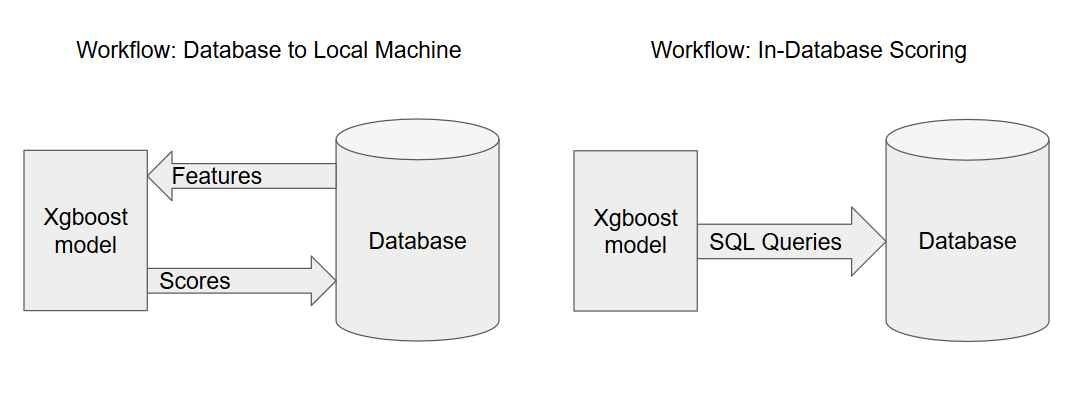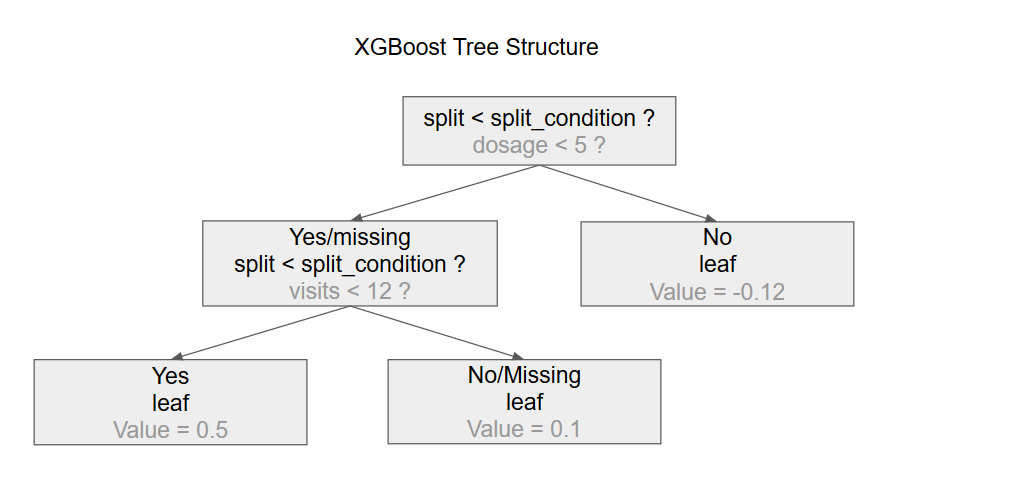# In-database xgboost predictions with R

by Roland Stevenson

Moving predictive machine learning algorithms into large-scale production environments can present many challenges. For example, problems arise when attempting to calculate prediction probabilities (“scores”) for many thousands of subjects using many thousands of features located on remote databases.

xgboost (docs), a popular algorithm for classification and regression, and the model of choice in many winning Kaggle competitions, is no exception. However, to run xgboost, the subject-features matrix must be loaded into memory, a cumbersome and expensive process.

Available solutions require using expensive high-memory machines, or implementing external memory across distributed machines (expensive and in beta). Both solutions still require transferring all feature data from the database to the local machine(s), loading it into memory, calculating the probabilities for the subjects, and then transferring the probabilities back to the database for storage. I have seen this take, 20-50 minutes for ~1MM subjects.

In this post, we will consider in-database scoring, a simple alternative for calculating batch predictions without having to transfer features stored in a database to the machine where the model is located. Instead, we will convert the model predictions into SQL commands and thereby transfer the scoring process to the database.We will convert the xgboost model prediction process into a SQL query, and thereby accomplish the same task while leveraging a cloud database’s scalability to efficiently calculate the predictions.

To accomplish this, we’ll need to work through a few steps. First, we’ll import the model as a list of nested tree structures that we can iterate through recursively. Then, we’ll create a function that will recursively descend through a tree and translate it into a SQL CASE statement. After that, we’ll create a query that sums the CASE statements for all trees before logit-transforming it to calculate a probability.

This first block of code loads the required packages and converts the model object to a list of trees that we can work with:

library(xgboost)
library(jsonlite)
library(whisker)

# our model exists in the variable xgb_model:
# dump the list of trees as JSON and import it as model_trees using jsonlite
model_trees <- jsonlite::fromJSON(
xgb.dump(xgb_model, with_stats = FALSE, dump_format='json'),
simplifyDataFrame = FALSE)Now, we need to translate each tree into a SQL CASE statement. Each tree represents a set of decisions based on whether a variable (the ‘split’) is less than a threshold value (the ‘split_condition’). The result of the decision could be ‘yes’, ‘no’, or ‘missing’. In each case, the tree provides the ‘node_id’ of the next decision to evaluate. When we reach a leaf, no decision needs to be made and instead a value is returned. An example tree is shown below:

We’ll also need a dictionary that maps an integer to its associated feature name, since the trees themselves refer to 0-indexed integers instead of the feature names. We can accomplish that by creating the following list:

feature_dict <- as.list(xgb_model$feature_names)  Using our feature_dict object, we can recursively descend through the tree and translate each node into a CASE statement, producing a sequence of nested CASE statements. The following function does just that: xgb_tree_sql <- function(tree, feature_dict, sig=5){ # split variables must exist to generate subquery for tree children sv <- c("split", "split_condition", "yes", "no", "missing", "children") # we have a leaf, just return the leaf value if("leaf" %in% names(tree)){ return(round(tree[['leaf']],sig)) } else if(all(sv %in% names(tree))){ tree$split_long <- feature_dict[[tree$split+1]] # +1 because xgboost is 0-indexed cs <- c(tree$yes, tree$no, tree$missing)
cd <- data.frame(
k = c(min(cs), max(cs)),
v = c(1,2)
)

tree$missing_sql <- xgb_tree_sql(tree$children[[cd$v[cd$k==tree$missing]]], feature_dict) tree$yes_sql <- xgb_tree_sql(tree$children[[cd$v[cd$k==tree$yes]]], feature_dict)
tree$no_sql <- xgb_tree_sql(tree$children[[cd$v[cd$k==tree$no]]], feature_dict) q <- " CASE WHEN {{{split_long}}} IS NULL THEN {{{missing_sql}}} WHEN {{{split_long}}} < {{{split_condition}}} THEN {{{yes_sql}}} ELSE {{{no_sql}}} END " return(whisker.render(q,tree)) } }  When we transform one tree into a sequence of nested CASE statements, we are producing a statement that yields that tree’s contribution to the total score. We now need to sum the output of each tree and then calculate the total probability prediction. In other words, we need to add up a list of nested CASE statements and then logit-transform the result. Note that below we make use of the R whisker package. This logic-less templating language is a great way to easily transform associative-arrays into SQL that contains easily identifiable labels as placeholders. We find this more readable than sequences of paste statements. xgb_sql_score_query <- function(list_of_trees, features_table, feature_dict, key_field = "id"){ # a swap list to render queries via whisker swap <- list( key_field = key_field, features_table = features_table ) # score_queries contains the score query for each tree in the list_of_trees score_queries <- lapply(list_of_trees, function(tree){ xgb_tree_sql(tree, feature_dict) }) # the query clause to sum the scores from each tree swap$sum_of_scores <- paste(score_queries, collapse=' + ')

# score query that logit-transforms the sum_of_scores
q <- "
SELECT
{{{key_field}}},
1/(1+exp(-1*( {{{sum_of_scores}}} ))) AS score
FROM {{{features_table}}}
"

return(whisker.render(q,swap))

}



We are now ready to generate the score query from our model:

queries <- xgb_sql_score_query(
model trees,
'mydataset.my_feature_table',
feature_dict
)

for(q in queries){
# example: run the query with the R bigrquery package
bq_project_query('my_project', q)
}


In summary, production models typically calculate predictions for all subjects on a daily, hourly, or even more frequent basis; however, moving feature data between a database and a local “scoring” machine is expensive and slow. Transferring the scoring calculations to run within the database, as we’ve shown above, can significantly reduce both cost and run time.

The astute reader may notice that, depending on the database, this will only work for a limited number of trees. When that becomes a problem, it is possible to add another layer that stores the summed scores for batches of trees as views or tables, and then aggregates their results. Beyond that, when queries with views become too long, it is possible to add an additional layer than aggregates batches of views into tables. We will save all of this for a future post.

Roland Stevenson is a data scientist and consultant who may be reached on Linkedin

Share Comments · · · ·